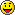# Common issues with circular coordinates

For a tube simulation I am doing I am working with circular coordinates. I.e. a point on the wall of the tube could be defined by two parameters: the point along the centerline (spline) of the tube, and the angular coordinate given in the range [0,1].

Because the angular coordinate 1 describes a full rotation, 1 = 0; (because 360 degrees will give the same result as 0 degrees rotation)

This leads to a couple of issues if we want to calculate the difference between two angular coordinates, or the mean between two angular coordinates.

Here are some functions that I use to calculate the mathematically correct modulo of 1, the correct distance between angular coordinates and the correct mean between angular coordinates. I don’t pride myself on my math so If anyone has a better method please let me know```	/// Mathematically correct modulo 1. This is the same as MyMath.Mod(a, 1.0f);
public static float Mod01(float a) {
return a - 1.0f * Mathf.Floor(a / 1.0f);
}

/// for numbers in the range [0,1] this will give the wraparound distance (i.e. (0,1) will give 0, (1,0.1) will give 0.1 etc.)
public static float WraparoundDistance01(float a, float b) {
float min = Mathf.Min (a,b);
float max = Mathf.Max (a,b);

float dist1 = max - min;
float dist2 = Mod01(min-max);

return Mathf.Min (dist1, dist2);
}

/// for numbers in the range [0,1] this will give the circular or wraparound mean. (i.e. (0.8,0.2) will give 0 instead of the arithmetical mean of 0.5)
public static float CircularAverage(float a, float b) {
float small = Mathf.Min (a,b);
float big = Mathf.Max (a,b);
float distance = WraparoundDistance01(small,big);
float diff = big - small;

float average;
if(diff > 0.5f) {
average = Mod01(big+distance*0.5f);
} else {
average = small + distance*0.5f;
}
return average;
}```